DATA PROBLEMS: Missing Data

By way of introducing the area of concern and organizing our thinking, consider the following table. The first row describes how it might come to pass that some of the data is missing in both cross section and time series data. If the data is missing for a reason that has nothing to do with the behavioral content of the model then the only consequence is that our estimators are less efficient. This is solely a result of sample size. In a cross section one could not predict which observations will be missing on the basis of some knowledge about the respondent pool, for example.
There is a class of problem in which observations are missing as a result of the process being modeled. This is the third row of the table. For example, we might be modeling the purchase of refrigerators. If the purchase price of a refrigerator is never below \$200, then in our sample we will never see a household that would have liked to purchase a refrigerator for \$175.

 Cross Section Time Series Nature of Problem Respondent fails to answer question Model needs monthly, we have quarterly Missing data unrelated to complete observations Lowers efficiency Lowers efficiency Gaps in data systematically related to behavior to be modeled See any text on censored and/or truncated data. The classic estimator for this genre is Tobit.

In the next table we categorize the missing data problems we can deal with at this point. Basically, you can be missing data for either the dependent variable or for some of the independent variables. The problem, or question, is how to leverage the data that one does have in order to increase the efficiency of the estimator.

 ya, xa na complete observations Case 1 ___, xb nb missing observations on yb Case 2 yc, ___ nc missing observations on xc

CASE 1 Missing observations for dependent variable
Let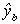be a predictor for yb so the least squares estimator for the coefficient vector in this filled data set is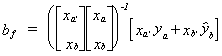. In general we can write the normal equations as x'y = x'x, so make this substitution for the data-on-hand estimator, ba, and the missing-data estimator, bb: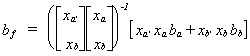.
Define F = [xa'xa + xb'xb]-1xa'xa so I - F = I - [xa'xa + xb'xb]-1xa'xa, or I-F = [xa'xa + xb'xb]-1xb'xb since I = [xa'xa + xb'xb]-1[xa'xa + xb'xb]. Then the filled data estimator is bf = Fba + (I-F)bb, with Ebf = Fb + (I-F)Ebb. Unbiasedness is seen to depend on Ebb.
Method 1
Let i be a column vector of ones. For the missing data estimator we will replace each missing observation on y by the mean of the observations on ya. Then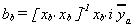where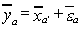and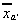is a row vector of variable means. Note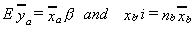where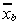is a column vector of variable means. Making the obvious substitution we get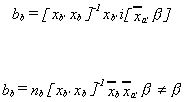Method 2
Get ba = (xa'xa)-1xa'ya then build b = xbba and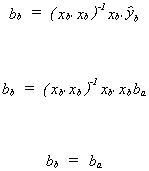Also,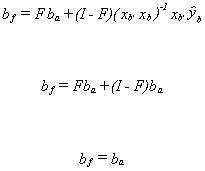Now for the variance. We have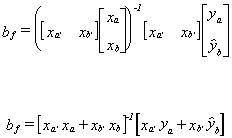Substitute in for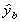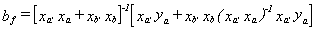factor out xa'ya and substitute in for ya

Post-multiplying bf by its transpose and taking expectations will give us the variance of our pooled estimator.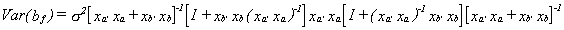If you multiply xa'xa through to the right then the last pair of square brackets cancel. Now factor (xa'xa)-1 out of the remaining terms and one is left with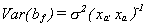. There is no gain in efficiency from using the filled estimator. In practice, because one must estimate s2, it will appear that the estimated standard errors of the filled estimator are smaller. Do you see why this is so? It depends only on the number of observations the computer uses in the calculated estimate of s2.

EXAMPLE: Left Hand Side Missing Data

Suppose that the entire correct data set is shown in the following table.

 Y x 0 0 2 1 1 2 3 1 1 0 3 3 4 4 2 2 1 2 2 1

If we use all of the observations to estimate a simple linear model then the result, with t-statistics in parentheses, is

 y = .8333 +.666x +e n=10 (1.75) (2.81)

But suppose that for some reason we do not observe the last four data points for y. Using the abbreviated data set our regression result is

 y = .6476 + .6341x +e n=6 (1.43) (1.55)

Using the coefficient estimates from this abbreviated database we construct fitted values for the last four observations on y, bringing our total number of observations back up to ten. Running the regression with 6 observed values for y and 4 fitted values for y gives us the following result

 y = .9268 +.6341x +e n=10 (2.32) (3.179)

Notice how much higher the t-statistics are than those in the regression when we had all of the correct data. This is contrary to the derived result. We had asserted above that our filled estimator was no more efficient. This is another case of the computer not really knowing what is going on. It must compute an estimate of the error variance from the observed data for use in an estimate of the variance of the coefficient estimator. In our filled data set the last four observations contribute nothing to the sum of squared errors, but the denominator is still 10. The consequence is that the estimate of the error variance is understated. The implication is that the careless researcher will use this filled estimator, which is no more efficient than the estimator based on the abbreviated data set, not question the very high observed t-statistics and reject too many null hypotheses.

Case 2 Missing observations on the right hand side.

Simple Regression
We have the simple regression model ya = a + bxa + ua and the additional nc observations on yc, but the observations on xc are missing. One possibility is to use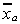to fill the blanks. This won't work since these terms would get zero weight in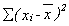. We would gain nothing for our effort.
Another possibility is to use the reverse regression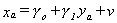to construct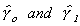then build a set of fitted values for xc according to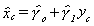. Now apply OLS to the filled model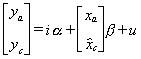. The sampling distribution of the OLS estimator for the parameter vector is unknown in this scheme so we can say nothing about unbiasedness and efficiency.

EXAMPLE: Missing data on the right hand side

For this example we use the same data as in the previous example. If we run the simple regression with y on the right hand side, x on the left hand side and using only the first six observations then we get the following result

 x = .7633 +.3817y +e n=6 (.24) (1.55)

Using these results to fill in for the missing values for x and applying OLS we get

 y = .7670 + .8685x +e n=10 (1.59) (2.90)

Notice that the slope estimate is higher than when we have all the data, as it was in the prior example. This is coincidence. It depends on the realization of the data which is available to us. That is, it is sample specific. Also, the t-statistic on the slope estimate is higher. This is not coincidence, for the same reason given in the earlier example.

Multiple Regression

Given the available data we have the following model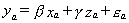(1)
for which we have na observations. We have another nc observations on yc and zc. From the additional observations we might fill in for the missing xc. We could use the first na observations on x and z in the following equation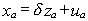(2)

In the last nc observations we would then substitute in for x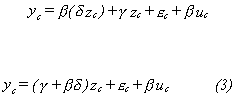The model then consists of the equations (1), (2), and (3). To operationalize

let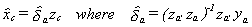.
Now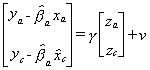. This method allows us to use more information about the dependent variable and z in our estimation of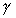. This should provide us with a more efficient estimator.

EXAMPLE: Right Hand Side Missing Data: Multiple Regression

We are given the following aggregate data for Taiwan. One could estimate a production function using this data

 YEAR CAPITAL LABOR OUTPUT 1958 17804. 275.50 16608. 1959 18097. 274.40 17511. 1960 18272. 269.70 20171. 1961 19167. 267.00 20933. 1962 19648. 267.80 20406. 1963 20804. 275.00 20832. 1964 22077. 283.00 24806. 1965 23445. 300.70 26466. 1966 24939. 307.50 27403. 1967 26714. 303.70 28629. 1968 29958. 304.70 29905. 1969 31586. 298.60 27508. 1970 33475. 295.50 29036. 1971 34822. 299.00. 29282. 1972 41794. 288.10 31536.

Using all of the data one obtains

 Q  = -1.6900 + 2.454L + .4326K + e (-2.97) (4.06) (5.48)

But suppose the last three observations on capital are missing. Using just the first twelve data points we observe

 Q = - 1.763 +2.1193 L +.5406 K + e (-1.76) (2.06) (2.39)

If we use the first 12 observations on capital and labor to estimate a linear regression the result is

 K = -2.926 + 3.8273 K + e (-3.35) (4.95)

The R2 is .71 and both coefficients are significant; L does quite a good job explaining capital. Use these coefficients to construct a fitted series for capital so that we have a total of 15 observations and run the original model again.

 Q = - 1.7610 + 2.4428 L + 5.406 K + e (-1.40) (1.58) (1.57)

The coefficient on labor does not change a great deal. Compared to the complete correct data set, the coefficient on K has changed by 25%, this is a big change. Compared to either the full or abbreviated data set the estimated coefficient standard errors have apparently gotten larger, the t-statistics have fallen.